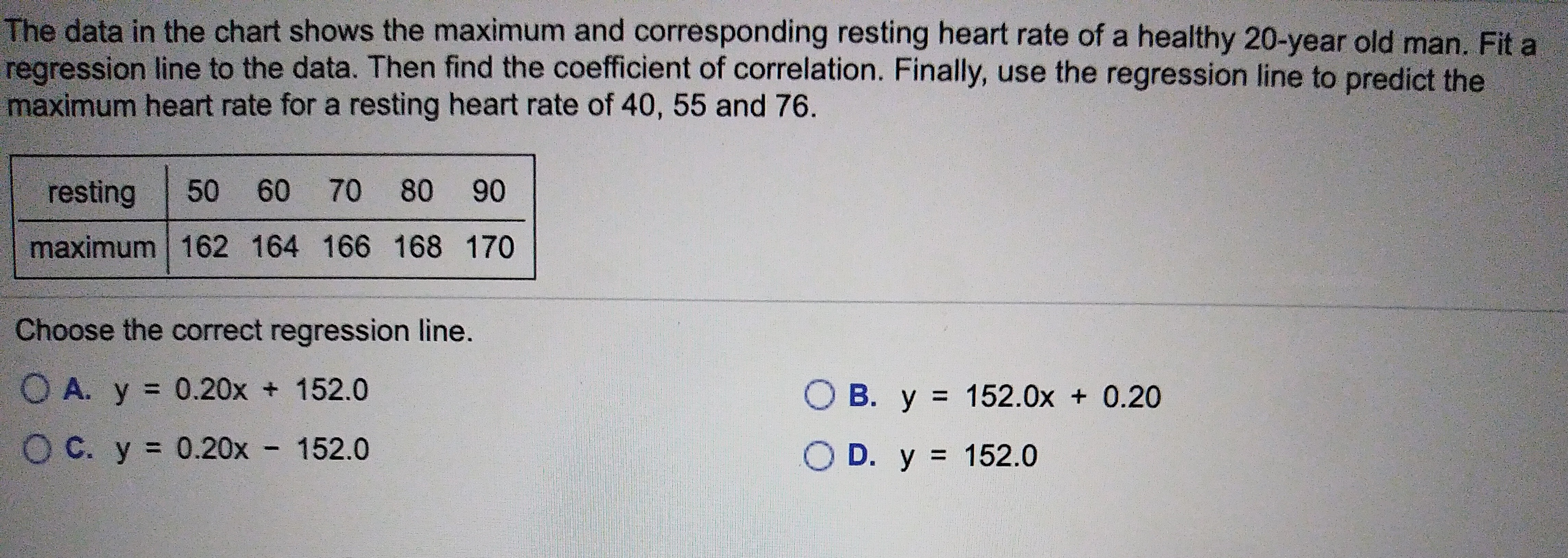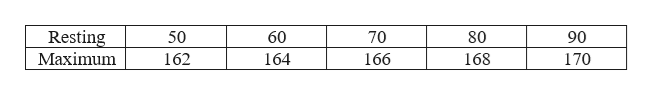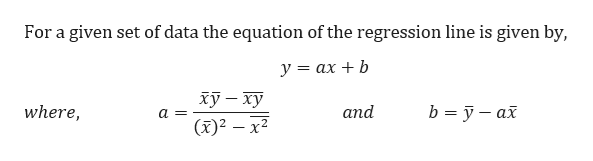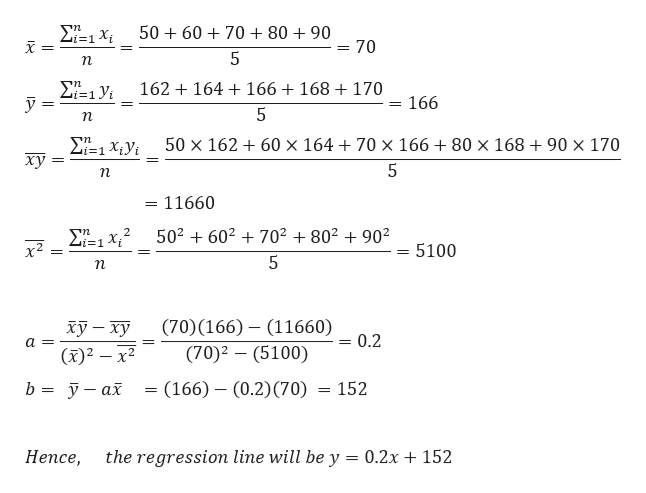The data in the chart shows the maximum and corresponding resting heart rate of a healthy 20-year old man. Fit aregression line to the data. Then find the coefficient of correlation. Finally, use the regression line to predict themaximum heart rate for a resting heart rate of 40, 55 and 76.60 70 8050resting90maximum 162 164 166 168 170Choose the correct regression line.O A. y 0.20x 152.0O B. y 152.0x 0.20O C. y 0.20x 152.0O D. y 152.0

Questionhelp_outlineImage TranscriptioncloseThe data in the chart shows the maximum and corresponding resting heart rate of a healthy 20-year old man. Fit a regression line to the data. Then find the coefficient of correlation. Finally, use the regression line to predict the maximum heart rate for a resting heart rate of 40, 55 and 76. 60 70 80 50 resting 90 maximum 162 164 166 168 170 Choose the correct regression line. O A. y 0.20x 152.0 O B. y 152.0x 0.20 O C. y 0.20x 152.0 O D. y 152.0 fullscreen
Step 1

The given data ishelp_outlineImage TranscriptioncloseResting Maximum 80 50 60 70 90 162 164 166 168 170 fullscreen
Step 2

We know that,help_outlineImage TranscriptioncloseFor a given set of data the equation of the regression line is given by, у %3 ах + b ху — ху (x)2 x2 where, and b yax a= fullscreen
Step 3

Let’s calculate a and b to...help_outlineImage TranscriptioncloseΣΥ-1 Χ. X 50 6070 80 + 90 = 70 5 n ΣΕ1 У 162 164166 + 168 + 170 i=1 166 5 n Σ-1xν. ху 50 x 162 60 x 164 +70 x 166 +80 x 168 + 90 x 170 i=1 5 n = 11660 Σ- x2 2 502 602702 802 902 i=1 5100 5 n (70)(166) (11660) (70)2 (5100) ху — ху (x)2 -x2 0.2 a= b%3D ў— ах = (166) - (0.2)(70) 152 Непсе, the regression line will be y = 0.2x152 Hence, fullscreen

Want to see the full answer?

See Solution

Want to see this answer and more?

Our solutions are written by experts, many with advanced degrees, and available 24/7

See Solution
Tagged in

Other